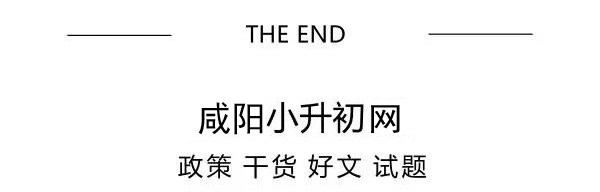# 小学奥数各年级经典题解题技巧大全——份数法（三）以份数法解变倍应用题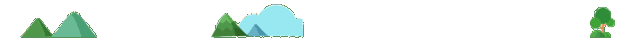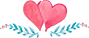*例1：

1300×3-1400

=3900-1400

=2500（千克）

2500-1300=1200（千克）

1200×3=3600（千克）*例2：

5×2=10（份）

10-1=9（份）

45÷9=5（人）

5×5=25（人）

25+15=40（人）

45÷（5×2-1）×5+15

=45÷9×5+15

=25+15

=40（人）（四）以份数法解按比例分配的应用题2331÷（24+21+18）=37（米）

37×24=888（米）…………………甲组任务

37×21=777（米）…………………乙组任务

37×18=666（米）…………………丙组任务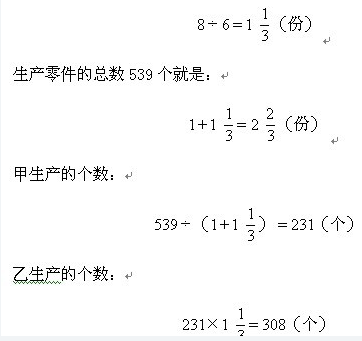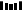ps：本文小编精心筛选，每天会不定时推送更多有关咸阳趣事、趣闻、学习资料、试卷分析等等推文，感兴趣，可以@小编哟

weixin  （linghangjy）

2018期末最全复习清单！（教师、学生、家长适用）

2019小升初【语、数、英】模拟卷+答案解析（可打印）